# Introduction to Algebra

A thorough introduction to Algebra for students in grades 6-9 who have completed our Pre-Algebra class. This course is designed to give students a strong foundation in the Algebra concepts that will be built on in subsequent courses. The curriculum focuses on inequality and system of equations; ratios, percent, and proportions; linear functions; factoring, quadratic equations, and functions; general functions, sequence, and series; polynomials, exponentials, and logarithms. Students will be prepared to tackle more advanced Algebra learning.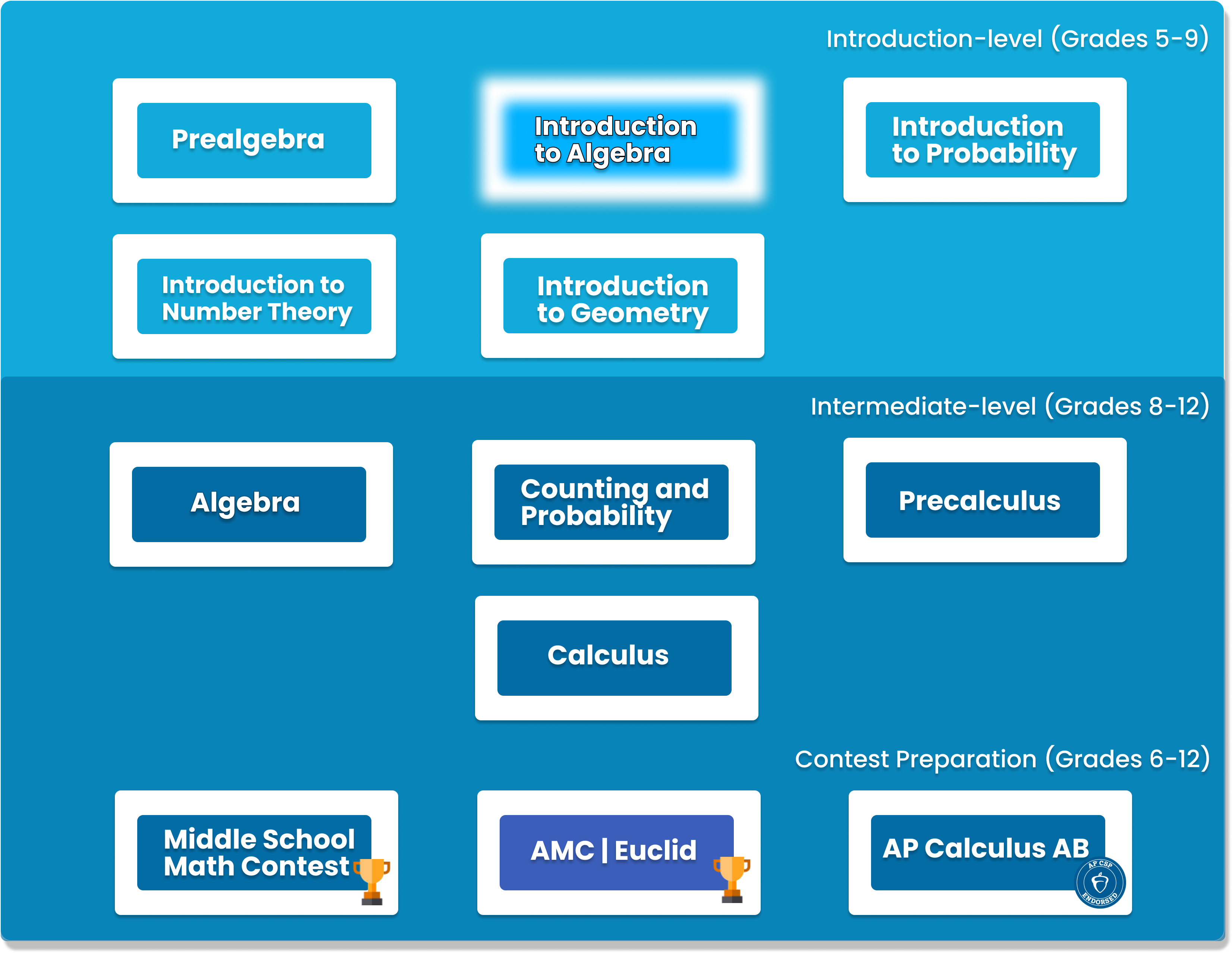###### Chapter 1: Follow the rulesReview of arithmetic, exponents and radicals
###### Chapter 2: X marks the spotsOperations on algebraic expressions (distribution, addition/subtraction)
###### Chapter 3: Linear EquationSolving linear equations and word problems
###### Chapter 4: More variablesOperations on algebraic expressions with more than one variable
###### Chapter 5: Multi-variable linear equationsSolving system of equations with two equations in two variables
###### Chapter 6: Ratios and PercentsChallenging problems on ratios and percents
###### Chapter 7: ProportionDirect, inverse, and joint proportions
###### Chapter 8: Graphing LinesProperties of the graph of the line y=ax+b
###### Chapter 9: Introduction to InequalitiesComparing two numbers, Linear Inequalities
###### Chapter 10: Quadratic Equations ISolving quadratic equations by factoring, Vieta’s formulae
###### Chapter 11: Special FactorizationsSquares and difference of squares, sum and difference of cubes, rationalizing denominators
###### Chapter 12: Quadratic Equations IISolving quadratic equations by completing the squares and using the quadratic formulaProperties of the graphs of parabolas y=ax^2+bx+c and circles x^2+y^2=r^2
###### Chapter 14: More InequalitiesQuadratic Inequalities, Trivial and AM-GM Inequality
###### Chapter 15: FunctionsDefinition of functions, composition and inverse of functions
###### Chapter 16: Graphing EquationsProperties of graphs of functions, transformation of functions
###### Chapter 17: PolynomialsAddition, subtraction, and multiplication of polynomials.
###### Chapter 18: Exponents and LogarithmsExponents, Logarithms, interest
###### Chapter 19: Special FunctionsRadicals, absolute value, floor and ceiling, rational functions, and piecewise functions
###### Chapter 20: Sequence and seriesArithmetic and geometric sequence and series
###### Chapter 21: Special ManipulationsMore techniques to solve equations by exploiting the self-similarity and symmetry of the problems

## Learn from the best

Passion for tech. Unmatched expertise. Personality that brings out the absolute best in your child. We know there's no replacement for the best teacher. It's no surprise that iconic companies like Google, Amazon, Microsoft, and Disney hire the same talent we do. Our Geek Certified instructors possess the whole package of skills that inspires students for the Tech World!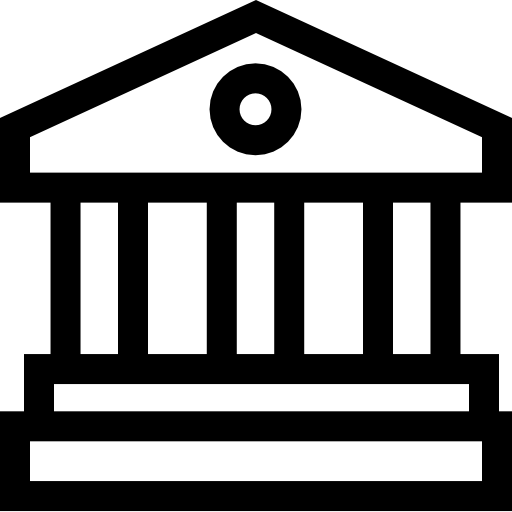Recruited from top universities and leading IT companies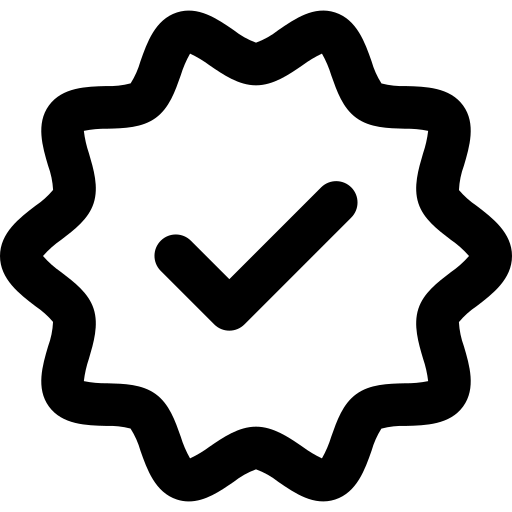Rigorous background and reference checks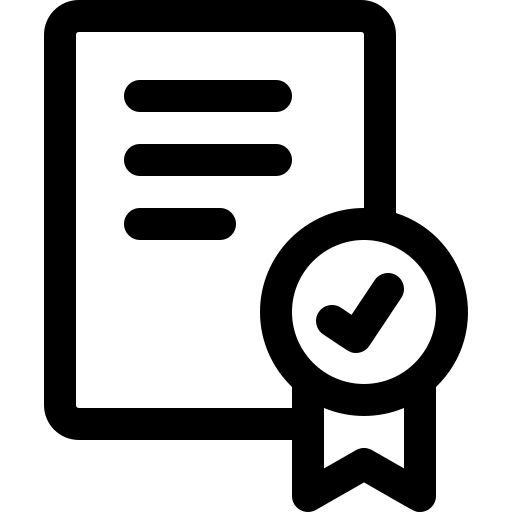Geekedu Certified professional level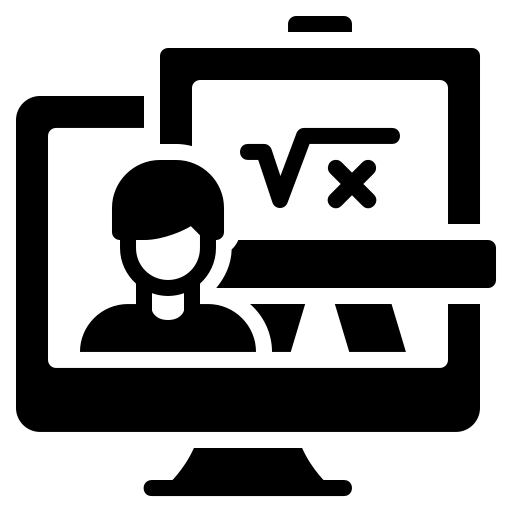Verified teaching experience

## What our parents say

This course is for students who have some previous beginner Algebra experience or have completed our Pre-Algebra course.Level:
AGES 10 - 13Online 1-on-1 Private Sessions

This course typically takes 3-6 months to complete. This depends on the student's experience and how fast they can master the concepts and the knowledge. Our instructors move at the pace of the student, it may take extra time for some students to reinforce what they have learned.

### You Might Also Be Interested In Our Elective Courses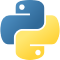##### Python Level 1
AGES 10 - 15

Designed for beginners, this level is designed to teach the basic fundamentals and design principles of Python, with the help of Turtle graphics and PyGame. This includes: variables, conditional statements, loop basics, and functions.

These concepts are transferable to any other programming language. Throughout the way, students will create projects in order to apply the concepts they have learned, and to solidify their knowledge.##### Python Level 2
AGES 10 - 15

Level 2 dives deeper into the basics of Python for a more thorough understanding and introduces advanced topics. Students will build on knowledge from Level 1 and work with data structures, advanced loops, algorithms, and object-oriented programming, and create games based on what they learn.

Students will complete the course with a solid understanding of Python fundamentals.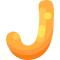##### Java Level 1
AGES 10 - 15

This beginner-friendly course serves as an introduction to the Java programming language. Students will learn the fundamentals of Java along with core computer science concepts.